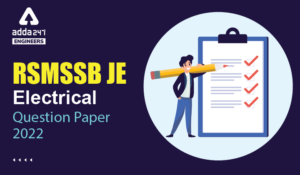Engineering Jobs   »   Civil Engineering Quiz

# RSMSSB-JE’21 CE: Daily Practices Quiz. 21-Aug-2021

Quiz: Civil Engineering
Exam: RSMSSB-JEn
Topic: Miscellaneous

Each question carries 1 mark
Negative marking: 1/3 mark
Time: 8 Minutes

Q1. An area of a field is 250 square centimeters from a shrunk map having shrunk factor of 0.9. Calculate the original area (Square meters) on the field, if the original scale of the map is 1 cm = 50m.
(a) 309
(b) 625000
(c) 694444
(d) 771600

Q2. A cohesive soil yields a maximum dry density of 16 kN/m³ during a standard proctor Compaction test. What would be its void ratio the specific gravity is 2.60 –
(a) 0.552
(b) 0.625
(c) 0.712
(d) 0.583

Q3. The pressure sustained per unit deformation of subgrade at specified deformation level using specified plate size is termed as
(a) CBR
(b) Safe bearing capacity
(c) Tangent modulus

Q4. In a Sutro weir, the discharge is proportional to
(a) H^(1/2)
(b) H^(3/2)
(c) H^(5/2)
(d) H^

Q5. The detention period of a septic tank is of the order of
(a) 2-6 hours
(b) 2-4 hours
(c) 12-36 hours
(d) 4-8 hours

Q6. As per IS:800-1984, what is the axial tension for mild steel power driven rivets?
(a) 100 Mpa
(b) 250 Mpa
(c) 415 Mpa
(d) 1000 Mpa

Solutions

S1. Ans.(d)
Sol. Measured Area of field on map = 250 cm²
Shrinkage factor (S.F) = 0.9
Original scale (S) = 1 cm = 50 m.
Original area of map =(Measured area )/(S.F.)^2
=250/(0.9)^2
=308.64 cm^2
Scale (S) = 1 cm = 50 m.
Hence, Area of field = 308.64 × (50)^2 = 771600 m²

S2. Ans.(b)
Sol. dry density (r_d )= 16kN/m³
G= 2.60
r_d=(Gr_w)/(1+e)
1+e=(2.60×9.81)/16
e=0.5941
▭(e=0.60)

S3. Ans.(d)
Sol. The pressure sustained per unit deformation of subgrade at specified deformation level using specified plate size is termed as modulus of subgrade reaction.
▭(Moduius of subgrade reaction (K)=(pressure (P))/(Deflection (D)))
(unit = kg/m³)
It is generally calculated at 0.125 cm or 1.25 mm.

S4. Ans. (d)
Sol. Relation for discharge over weir

Q ∝ H^n

n = 3/2 for Reatangular weir
n = 5/2 for triangular weir
n = 1 for Proportional or Sutro weir

S5. Ans.(c)
Sol. The detention period of septic tank is 12 to 36 hours.

S6. Ans.(a)
Sol. Maximum permissible stress.

 Axial tension Shearing Bearing Power driven Shop rivet 100 100 300 Hand driver shop rivet 80 80 250

Sharing is caring!

### TOPICS:

•RSMSSB JE Exam Pattern 2023, Check Lates...
•RSMSSB JE 2022 DV Schedule, Download RSM...
•RSMSSB JE Result 2022, OUT-Direct Link t...
•RSMSSB Computer Instructor Exam date 202...
•RSMSSB JE Electrical Question Paper 2022...
•RSMSSB JE Civil Question Paper 2022, Che...